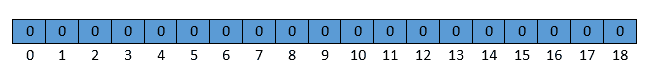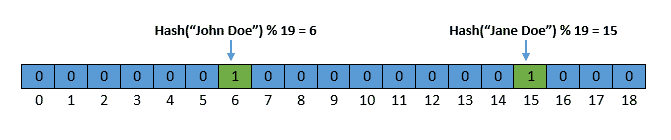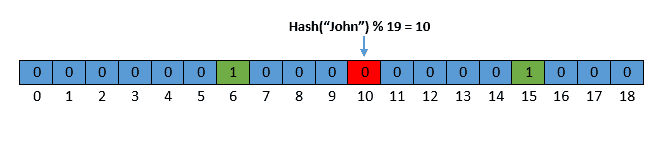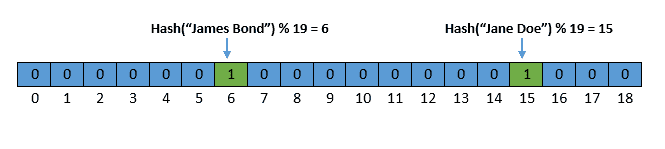## 1. Overview

I’m sure many of us must have seen the warning message – the username or e-mail is already taken. If we notice carefully, the warning message appears within a few seconds. All websites perform this validation during the sign-up process.

Have we ever wondered how websites validate millions of records within seconds? One of the solutions is using the Bloom filter

In this tutorial, we’ll discuss what a Bloom filter is, its supported operations, usage, and limitations.

## 2. What Is a Bloom Filter?

Bloom filter is a probabilistic data structure. It’s used to test whether an element is a member of a set. Of course, one can achieve the same result using other data structures as well. However, the Bloom filter does this in space and time-efficient way.

Let’s understand how the Bloom filter is implemented. Under the hood, the Bloom filter is just an array of bits with all bits set to zero initially. The below diagram depicts the Bloom filter of size 19:## 3. Bloom Filter Operations

Bloom filter supports only two operations, Insert and Lookup. Let’s understand them with the example:

### 3.1. Insert

We can perform Insert operations in constant space and time complexity.  Bloom filter performs the below steps for Insert operation:

1. Hash the input value
2. Mod the result by the length of the array
3. Set corresponding bit to 1

Let’s illustrate this by inserting two strings – John Doe and Jane Doe into the Bloom filter. Let’s assume that hash values for these two strings are 1355 and 8337, respectively. Now, let’s perform the modulo operation to get an index within the bounds of the bit array: 1355%19 = 6 and 8337%19 = 15.

The Bloom filter will look like this after inserting values:### 3.2. Lookup

We can perform a Lookup operation in constant time complexity. Bloom filter performs the below steps as a part of the Lookup operation:

1. Hash the input value
2. Mod the result by the length of the array
3. Check if the corresponding bit is 0 or 1

If the bit is 0, then that input definitely isn’t a member of the set. But if the bit is 1, then that input might be a member of a set. So let’s check if string John is present or not in the Bloom filter:In this example, the bit at the 10th index is 0, which indicates that the given string isn’t present in the Bloom filter.

## 4. False Positive Analysis

Bloom filter is a space and time-efficient data structure. However, the tradeoff for that efficiency is that it’s probabilistic in nature. It means that searching for a nonexistent element can give an incorrect answer. In this section, we’ll understand a false-positive scenario and a possible solution to reduce its frequency.

Let’s check if the string James Bond is present or not in the Bloom filter. Assume that the hash value of the input string is 1355, which is the same as John Doe.In this example, the Lookup operation returns the true result. However, we never inserted the string James Bond in the Bloom filter.

The false-positive scenario occurs due to hash collision. We can use multiple hash functions to reduce the hash collision frequency. So instead of setting only one bit, multiple bits will be set for a single input.

## 5. Applications of the Bloom Filter

Bloom filters are used by many popular applications due to their efficiency. Let’s discuss some of their use cases:

• Spell Checker: In the early days, spell checkers were implemented using the Bloom filter
• Databases: Many popular databases use Bloom filters to reduce the costly disk lookups for non-existent rows or columns. This technique is used by PostgreSQL, Apache Cassandra, Cloud Bigtable, etc
• Search Engines: BitFunnel is a search engine indexing algorithm. It uses the Bloom filter for its search indexes
• Security: Bloom filters are used to detect weak passwords, malicious URLs, etc

## 6. Limitations of the Bloom Filter

Though the Bloom filter is a space and time-efficient data structure, there are a few limitations of it:

• The naive implementation of the Bloom filter doesn’t support the delete operation
• The false-positives rate can be reduced but can’t be reduced to zero

## 7. Conclusion

In this article, we discussed what a Bloom filter is and its supported operations. Then we saw the false-positive nature of the Bloom filter and the possible solution to make it less error-prone. We also discussed how some of the popular software leverages the Bloom filter to improve its efficiency. Finally, we looked at the limitations of the Bloom filter.